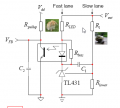#### mishra87

Joined Jan 17, 2016
908
Can anybody help me out on my design black box design ???Lets given -
Vout = 5V
Vdd = 5V
Vref_TL431 =2.5V
TL817_CTR_ Standard one can assume 130% or as per datasheet
Vf_Led =1.4V

Now how do we calculate -
Detail explanations are welcome.
R1 = ?
Rlower=?
Rled=?
Rbias=?
C1=?
Rpullup=?
C2=?
Vfb=?

I need to understand black box calculation for each component >>

Thank you so much in Advance !!!

#### mishra87

Joined Jan 17, 2016
908
Attention needed in this post.

#### crutschow

Joined Mar 14, 2008
27,727
R1 = ?
Rlower=?

For regulating to a Vout of 5V the voltage divider of R1 and Rlower needs to generate 2.5V at the input to the TL431 (which has a maximum input current of 4μA).
This is achieved with equal value resistors.
A value of 1k for each draws little current from the output but is still low enough that the feedback voltage accuracy is not significantly affected by the 4μA of current through the 500Ω equivalent resistor of R1 and Rlower at the TL431 input.
(4μA through 500Ω gives a 2mV change in voltage)

Rled=?
The TL431 needs a minimum of 1 mA of bias current for operation, and allowing another 1 mA for signal means 2 mA through Rled.
The Opto LED voltage is 1.2V and, allowing 2.5V across the TL431, the voltage drop across Rled is 5V - 1.2V - 2.5V = 1.3V.
The maximum resistance for Rled is then 1.3V / 2mA = 650Ω.
To allow for component tolerances I would make it no more than 500Ω.

Rbias=?
To maintain a minimum of 1mA through the TL431 when the Opto LED is fully off, Rbias = 1.2 / 1mA = 1.2kΩ maximum.
For tolerance considerations I would make it no more than 1kΩ.

C1=?
That value must be selected to help stabilize the loop, and that can only be determined when all the other parts in the loop are connected.

Rpullup=?
That must be selected to give the Vfb you need at about 0.5mA of bias current from the opto output.

C2=?
See C1

Vfb=?
That voltage is determined by the rest of the circuit in the loop

Last edited:
•mishra87

#### DickCappels

Joined Aug 21, 2008
7,707
What is the objective of your project, or is this homework?

#### mishra87

Joined Jan 17, 2016
908
Thank you so much for your valuable time and information.

It's only black box calculation before actual product desiging.!!!

Now can anybody discuss about loop stability and calculations of it.

Thank you so much again !!!

#### mishra87

Joined Jan 17, 2016
908
R1 = ?
Rlower=?

For regulating to a Vout of 5V the voltage divider of R1 and Rlower needs to generate 2.5V at the input to the TL431 (which has a maximum input current of 4μA).
This is achieved with equal value resistors.
A value of 1k for each draws little current from the output but is still low enough that the feedback voltage accuracy is not significantly affected by the 4μA of current through the 500Ω equivalent resistor of R1 and Rlower at the TL431 input.
(4μA through 500Ω gives a 2mV change in voltage)

Rled=?
The TL431 needs a minimum of 1 mA of bias current for operation, and allowing another 1 mA for signal means 2 mA through Rled.
The Opto LED voltage is 1.2V and, allowing 2.5V across the TL431, the voltage drop across Rled is 5V - 1.2V - 2.5V = 1.3V.
The maximum resistance for Rled is then 1.3V / 2mA = 650Ω.
To allow for component tolerances I would make it no more than 500Ω.

Rbias=?
To maintain a minimum of 1mA through the TL431 when the Opto LED is fully off, Rbias = 1.2 / 1mA = 1.2kΩ maximum.
For tolerance considerations I would make it no more than 1kΩ.

C1=?
That value must be selected to help stabilize the loop, and that can only be determined when all the other parts in the loop are connected.

Rpullup=?
That must be selected to give the Vfb you need at about 0.5mA of bias current from the opto output.

C2=?
See C1

Vfb=?
That voltage is determined by the rest of the circuit in the loop
What about the CTR value given in opto datasheet. Is there any impact of Rpullup calculation.

#### Dodgydave

Joined Jun 22, 2012
10,050
Vout for 5V R1 and lower are the same value, like 10K each, for Rled try 330R, C1 100nF.

•mishra87

#### crutschow

Joined Mar 14, 2008
27,727
Now can anybody discuss about loop stability and calculations of it.
What about the CTR value given in opto datasheet. Is there any impact of Rpullup calculation.
Yes, of course.
In your first post you said to use 130%.
For worst-case design you should use the minimum CTR value.

•mishra87

#### mishra87

Joined Jan 17, 2016
908
Vout for 5V R1 and lower are the same value, like 10K each, for Rled try 330R, C1 100nF.
Thank you so much your time .

Actually I need theoretical calculation for all these value because everywherr in datasheet and application note values are written.

Loop stability by using-

Type -1 compensation -where to use this one.
Type - 2 compensation -where to use this one.
Type 3 compensation -where to use this one.

How to calculate these values. !!!

Yes, of course.
In your first post you said to use 130%.
For worst-case design you should use the minimum CTR value.
Thank you so much for your time. !!!

Loop stability of SMPS. !!!

Last edited by a moderator:

#### mishra87

Joined Jan 17, 2016
908
Could anybody let me know that in this circuit which mode of operation are doing.
Voltage mode control of feedback or current mode control of feedback. !!!
What is difference between these two mode of operation.